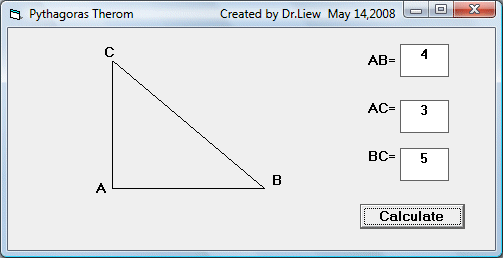# Pythagoras Theorem

This a program that can solve geometric problems based on Pythagorean Theorem.  We suppose everybody is already familiar with the above Theorem. However, some of you might have forgotten this theorem. So, let us list the formula here. By referring to a right angled triangle ABC, if the sides are AB, AC and AC respectively, where AC  is the hypotenuse, then AB, AC and BC are connected by the formula      AB2+AC2=BC2

Using the above formula, you can calculate the third side if the the length of any two sides are know. For example, if AB=4 and AC=3 then BC=5. We have designed the VB program for the user to input any two sides and then he or she is able to calculate the third side automatically. The third side BC can be found by finding the square root of AB2+AC2 . In visual basic, the syntax is

BC= Sqr(AB ^ 2 + AC ^ 2)

### The Interface### The Code

Private Sub Command1_Click()

Dim AB, AC, BC As Single
AB = Val(Txt_AB.Text)
AC = Val(Txt_AC.Text)
BC = Val(Txt_BC.Text)
If AB <> 0 And AC <> 0 Then
BC = Sqr(AB ^ 2 + AC ^ 2)
Txt_BC.Text = Round(BC, 2)
ElseIf AB <> 0 And BC <> 0 Then
AC = Sqr(BC ^ 2 - AB ^ 2)
Txt_AC.Text = Round(AC, 2)
ElseIf AC <> 0 And BC <> 0 Then
AB = Sqr(BC ^ 2 - AC ^ 2)
Txt_AB.Text = Round(AB, 2)
End If

End Sub